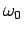Next: Acknowledgments Up: 4. Spectral Properties of Previous: 4.4 Discussion

# 4.5 Conclusions

In this chapter we study the spectral properties of the one-dimensional Holstein and breathing polaron models. While the H model has a momentum independent electron-phonon coupling, the B coupling is a monotonic increasing function of the phonon momentum. We find the renormalization of the quasiparticle properties at small momentum in the B model weaker than that in the H one for a dimensionless coupling constant defined as the ratio of the lattice deformation energy and the kinetic energy of the electron. With increasing phonon frequency, the B quasiparticle weight decreases in contrast to the H case where it increases. The quasiparticle dispersion in the H model displays a kink and a small gap at an excitation energy equal to. On the other hand, the B model dispersion, besides the gap at, exhibits another, more pronounced one at energy. This is due to the following facts: the momentumof the relevant phonons in the scattering process increases with increasing the total polaron momentum and that the B coupling is an increasing function of. As a consequence the renormalization of the B polaron increases with increasing polaron momentum. However the first fact is dependent on the free electron dispersion and does not hold if it has low energy states separated by large momentum. In that case the difference between the B and the H polaron becomes less significant.

The specific momentum dependence of the B bare electron-phonon coupling results in an effective coupling which is an increasing function of the total polaron momentum, such that the small momentum polaron properties are weak coupling while the large momentum ones are strong coupling. This might be relevant for explaining the peculiar behavior of the high energy quasiparticle dispersion (5) or the temperature dependency of the photoemission linewidth (24) in cuprate superconductors.Next: Acknowledgments Up: 4. Spectral Properties of Previous: 4.4 Discussion
© Cyrill Slezak